×

Search anything:

# 4 Types of Machine Learning

#### Machine Learning (ML)Get this book -> Problems on Array: For Interviews and Competitive Programming

In this article, we have explored the 4 different types of Machine Learning (ML). The goal of ML is to create techniques so that computers can act close to human behaviour and the current techniques fall into 4 distinct categories.

Before we dive into Types of Machine Learning, Let us Take a quick detour to understand what is Machine Learning?.

# What is Machine Learning?

Before Asking what is Machine Learning? Let us first understand what are computers. Computers are machines that can be programmed to perform a set of instructions. Computers are Good at Doing Recurrent Works. The Usual Way to Make useful work is to have clever human programmers write the instructions for the computer to follow.But, this is very tedious work and it is very hard to write Rules for Everything. So, we need a way to make computers learn from data. This is where Machine Learning Comes in to Action.

Machine learning is a new paradigm in computer science,let us understand how it is different from any traditional programming.

Let us see how traditional programming works.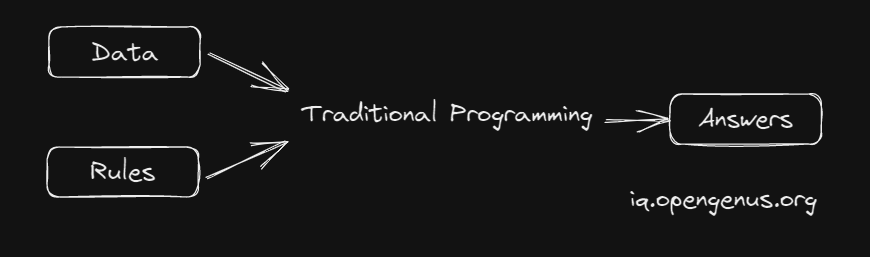In the Above Picture we can see that we have Rules and Data as Inputs and Answers as Output. We have to write a program that takes Rules and Data as Input and gives Answers as Output. This is called Traditional Programming.

Now, Let us see how Machine Learning Works.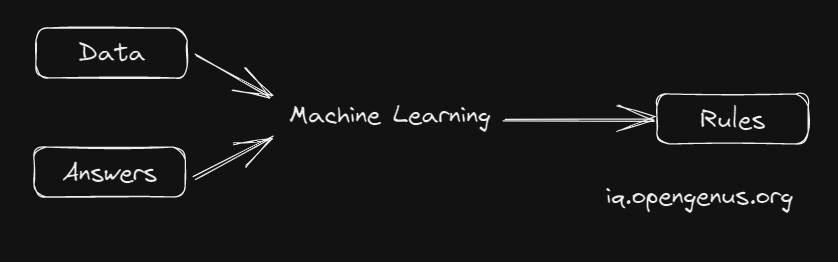In the Above Picture we can see that we have Data and Answers as Inputs and Rules as Output. We have to write a program that takes Data and Answers as Input and gives Rules as Output. This is called Machine Learning.

With This we Understood What is Machine Learning. Now, Let us see the Types of Machine Learning.

# Types of Machine Learning

Machine Learning can be broadly classified into 4 types.

1. Supervised Learning
2. Unsupervised Learning
3. Reinforcement Learning
4. Semi-Supervised Learning

# Supervised Learning

Supervised learning is a type of machine learning that involves training a model on a labeled dataset.what is labeled dataset? A labeled dataset is a dataset that contains both the input and the output. The model is trained in the Input dataset to produce Output which is Compared with the Actual Output. The model is then trained again to produce better results. This process is repeated until the model is able to produce the desired output.

Superivsed Learning can be further classified into 2 types.

1. Regression
2. Classification

## Regression

Regression is a type of Supervised Learning in which the output is a continuous value. For Example, Predicting the Price of a House, Predicting the Temperature, Predicting the Stock Price, etc.

## Classification

Classification is a type of Supervised Learning in which the output is a Categorical Value. For Example, Identify a fruit, Identify a person, Identify a type of flower, etc.

Ok, We Have Seen what is SuperVised Learning and How it is Implemented.

### Types of Supervised Learning Algorithms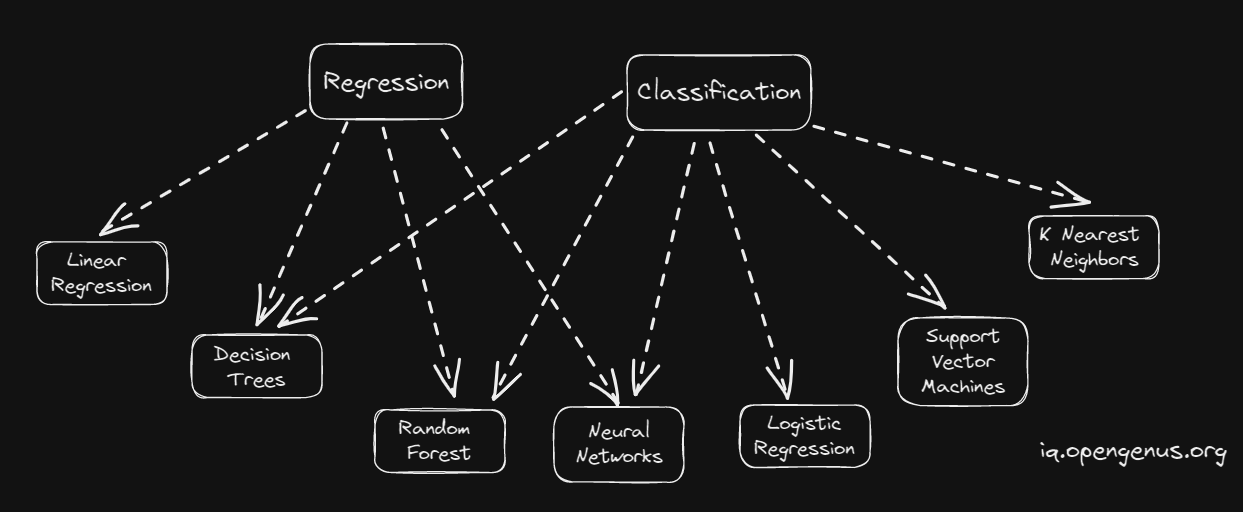As we Can see Few Algorithms are common for both Regression and Classification.

## Code Implementation

Now,Let us Take SVM Algorithm to See the Process of Supervised Learning

### Data

Here we have Created a Sample Data using Make_Blobs

``````
import numpy as np
import matplotlib.pyplot as plt
from sklearn import svm, datasets
from sklearn.datasets import make_blobs

n_samples = 1000
centers = 3
(X, y) = make_blobs(n_samples=n_samples, n_features=2,
centers=centers, cluster_std=1.05, random_state=40)

``````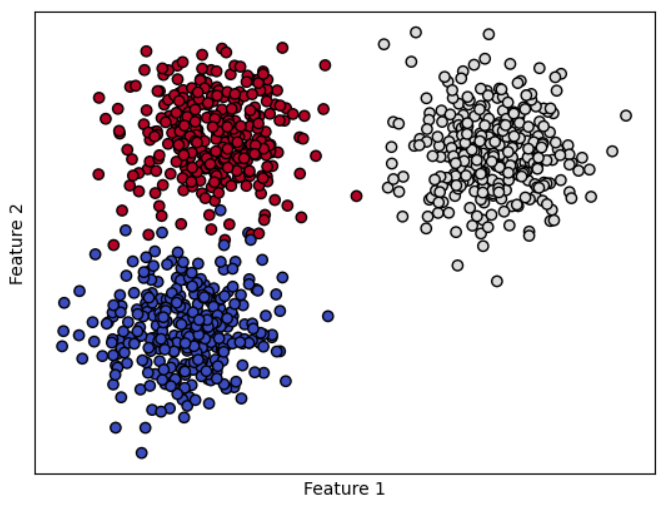``````def make_meshgrid(x, y, h=0.02):
x_min, x_max = x.min() - 1, x.max() + 1
y_min, y_max = y.min() - 1, y.max() + 1
xx, yy = np.meshgrid(np.arange(x_min, x_max, h),
np.arange(y_min, y_max, h))
return xx, yy

def plot_contours(ax, clf, xx, yy, **params):
Z = clf.predict(np.c_[xx.ravel(), yy.ravel()])
Z = Z.reshape(xx.shape)
return ax.contourf(xx, yy, Z, **params)

models = svm.SVC(kernel="linear", C=0.1)
clf = models.fit(X, y)

# title for the plots
titles = "SVM with Linear Kernel"

# Set-up 2x2 grid for plotting.
fig, ax = plt.subplots()

X0, X1 = X[:, 0], X[:, 1]
xx, yy = make_meshgrid(X0, X1)

# for clf, title, ax in zip(models, titles, sub.flatten()):
plot_contours(ax, clf, xx, yy, cmap=plt.cm.coolwarm, alpha=0.8)
ax.scatter(X0, X1, c=y, cmap=plt.cm.coolwarm, s=20, edgecolors="k")
ax.set_xlim(xx.min(), xx.max())
ax.set_ylim(yy.min(), yy.max())
ax.set_xlabel("Feature 1")
ax.set_ylabel("Feature 2")
ax.set_xticks(())
ax.set_yticks(())
ax.set_title(titles)
plt.show()
``````

### Results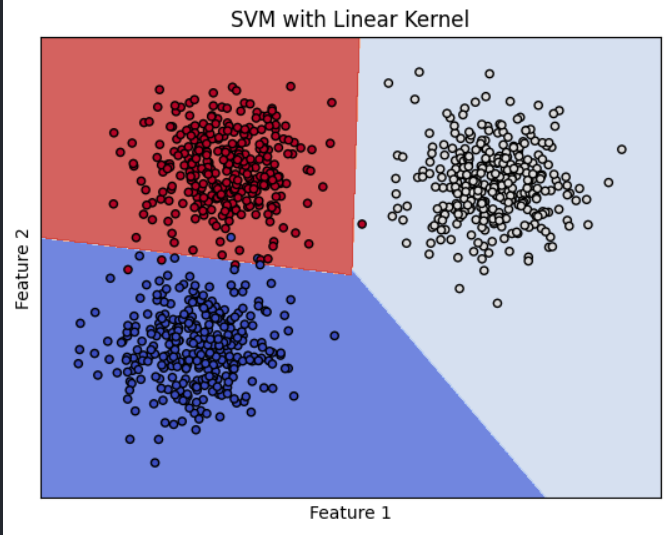As we can from Results that Our SVM Classifier Able to Classify the Data Correctly.In Further Section we will see how we can classify the same data with unsupervised Algorithm.

We had seen what is Supervised Learning and How it is Implemented. Now, Let us see what is Unsupervised Learning.

# Unsupervised Learning

Unsupervised learning is a type of machine learning that involves training a model on an unlabeled dataset. What is an unlabeled dataset? An unlabeled dataset is a dataset that contains only the input and not the output. The model is trained in the Input dataset to produce Output which is Compared with the Actual Output. The model is then trained again to produce better results. This process is repeated until the model is able to produce the desired output.

Unsupervised Learning can be further classified into 2 types.

1. Clustering
2. Association

## Clustering

Clustering is a type of Unsupervised Learning in which the output is a Categorical Value. For Example, Grouping of similar fruits, Grouping of similar people, Grouping of similar flowers, etc.

## Association

Association is a type of Unsupervised Learning in which the output is a Categorical Value. For Example, Finding the association between fruits and people, Finding the association between flowers and people, etc.

Ok, We Have Seen what is UnSuperVised Learning and How it is Implemented.

### Types of Unsupervised Learning Algorithms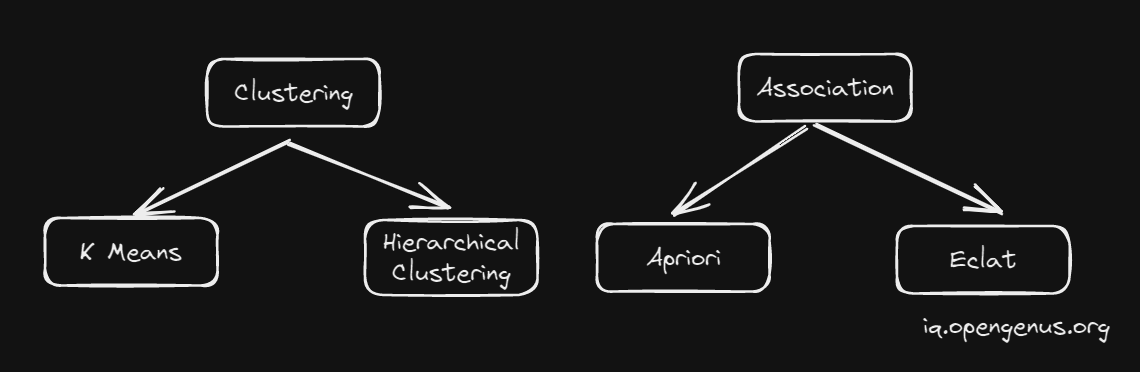## Code Implementation

we will be using K-means Algorithm for Classifying the Data

``````from sklearn.cluster import KMeans

kmeans = KMeans(n_clusters=3, random_state=0).fit(X)
y_kmeans = kmeans.predict(X)

plt.scatter(X[:, 0], X[:, 1], c=y_kmeans, s=50, cmap='viridis')
centers = kmeans.cluster_centers_
plt.scatter(centers[:, 0], centers[:, 1], c='black', s=20,edgecolors="k");
plt.title('kmeans clustering')
plt.xlabel("Feature 1")
plt.ylabel("Feature 2")
plt.show()

``````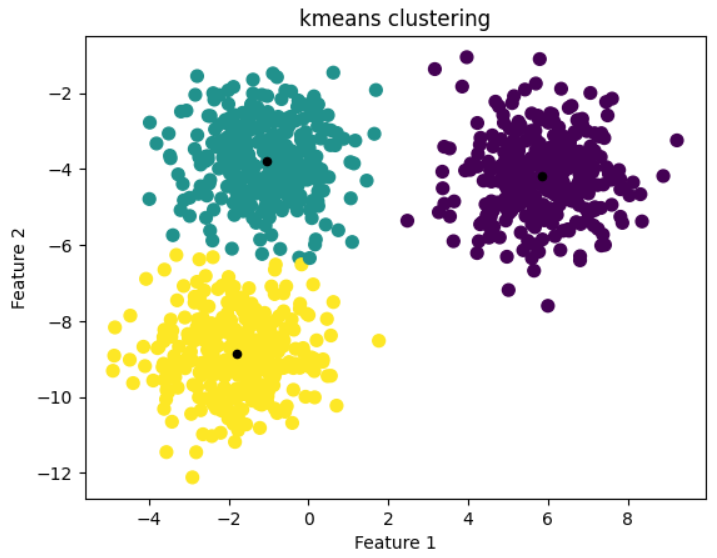From Results Above We can See our Model able to Classify the Most of the Data Correctly.

We had seen what is Unsupervised Learning and How it is Implemented. Now, Let us see what is Reinforcement Learning.

# Reinforcement Learning

Reinforcement Learning is a type of machine learning where it involves training a model to take actions based on states in environment to Maximize it Cumulative Reward.In Reinforcement Learning, the model is not given any explicit input-output pairs to learn from. Instead, the model is given a reward for each action it takes in a given state. The model is then trained to maximize the cumulative reward.

Out all the Types of Machine Learning, Reinforcement Learning is the most related to Natural Intelligence. Reinforcement Learning is used in Self Driving Cars, Playing Games, etc.

Let us Look at an Example of Reinforcement Learning.

Imagine a person training his dog by throwing a stick. The dog needs to catch the stick. The person is the environment, dog is the agent. The action is that dog has to catch the stick, reward is the stick that the dog catches and the path of the stick is the observation.

We can see dog owner is training his dog to fetch a Stick. The dog is given a reward for fetching the Stick. The dog is then trained to maximize the cumulative reward.

Ok, We Have Seen what is Reinforcement Learning and How it is Implemented.

In Reinforcement we have 4 types of Algorithms.

1. Q-Learning
2. Deep Q-Learning
3. SARSA
4. DDPG

We had Seen all Major Types of Machine Learning. Now, Let us see what is Semi-Supervised Learning.

# Semi-Supervised Learning

What is Semi-Supervised Learning? Semi-Supervised Learning is a type of Machine Learning that involves training a model on a partially labeled dataset. What is a partially labeled dataset? A partially labeled dataset is a dataset that contains both the input and the output. But, the output is not completely labeled. The model is trained in the Input dataset to produce Output which is Compared with the Actual Output. The model is then trained again to produce better results. This process is repeated until the model is able to produce the desired output.

Semi-Supervised Learning bridges the gap between Supervised Learning and Unsupervised Learning. It is used in Image Classification, Text Classification, etc. It uses Less Labelled Data Compared to Supervised Learning.

## Trianing a Model on a Partially Labeled Dataset

The Easiest Way is Self Training,

Let us How it is Triained, Steps are as Follows.

1. Train a Model on a Labeled Dataset.
2. Use the Model to Predict the Labels for the Unlabeled Dataset,Predicted Labels are called Pseudo Labels.
3. Train a Model on the Labeled Dataset and the Predicted Labels for the Unlabeled Dataset.
4. Repeat Step 2 and 3 until the Model is able to produce the desired output.

Let us Visualize Training Process,# Conclusion

In This Article we have Discussed What is Machine Learning and How it is Implemented. We have also Discussed the Types of Machine Learning. We have also Discussed the Types of Algorithms used in Machine Learning. We have also Discussed What is Semi-Supervised Learning and How it is Implemented.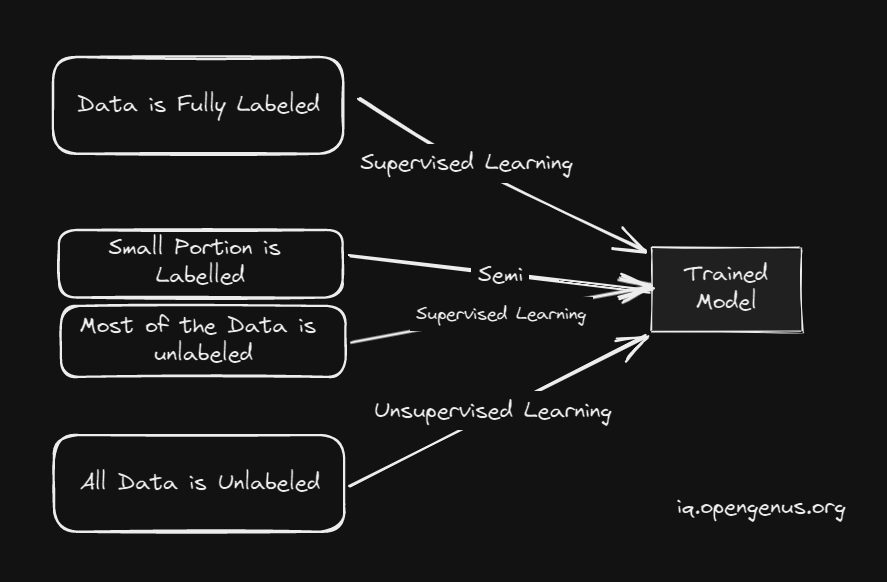## Question

#### Which on the Following is Supervised Algorithm

SVM
Kmeans
Q-Learning
Self-Training
Because,SVM requires Labelled Data

I hope you enjoyed the complete journey of Type of Machine Learning.